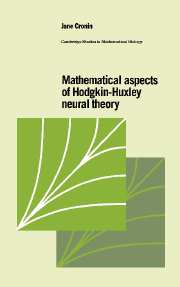Home > Catalog > Mathematical Aspects of Hodgkin-Huxley Neural TheorySearch this bookDetails

• Page extent: 276 pages
• Size: 216 x 138 mm
• Weight: 0.481 kg

Library of Congress

• Dewey number: 599/.0188
• Dewey version: 19
• LC Classification: QP363 .C76 1987
• Neural conduction--Mathematical models
• Purkinje cells--Mathematical models
• Electrophysiology--Mathematical models

Library of Congress Record

Hardback

(ISBN-13: 9780521334822 | ISBN-10: 0521334829)

Manufactured on demand: supplied direct from the printer

\$125.00 (C)

Professor Cronin offers, for the first time, a synthesis of the work of Hodgkin and Huxley on nerve conduction. This information has previously been scattered throughout various media and difficult to retrieve. In this book the author thoroughly describes and analyzes their mathematical models (nonlinear ordinary and partial differential equations) and later models.

Contents

1. Introduction; 2. Nerve conduction: the work of Hodgkin and Huxley; 3. Nerve conduction: other mathematical models; 4. Models of other electrically excitable cells; 5. Mathematical theory; 6. Mathematical analysis of physiological models; Appendix; References.